# E8 (mathematics)

In mathematics, E8 is any of several closely related exceptional simple Lie groups, linear algebraic groups or Lie algebras of dimension 248; the same notation is used for the corresponding root lattice, which has rank 8. The designation E8 comes from the Cartan–Killing classification of the complex simple Lie algebras, which fall into four infinite series labeled An, Bn, Cn, Dn, and five exceptional cases labeled E6, E7, E8, F4, and G2. The E8 algebra is the largest and most complicated of these exceptional cases.

Wilhelm Killing (1888a, 1888b, 1889, 1890) discovered the complex Lie algebra E8 during his classification of simple compact Lie algebras, though he did not prove its existence, which was first shown by Élie Cartan. Cartan determined that a complex simple Lie algebra of type E8 admits three real forms. Each of them gives rise to a simple Lie group of dimension 248, exactly one of which is compact. Chevalley (1955) introduced algebraic groups and Lie algebras of type E8 over other fields: for example, in the case of finite fields they lead to an infinite family of finite simple groups of Lie type.

## Basic description

The Lie group E8 has dimension 248. Its rank, which is the dimension of its maximal torus, is 8.

Therefore, the vectors of the root system are in eight-dimensional Euclidean space: they are described explicitly later in this article. The Weyl group of E8, which is the group of symmetries of the maximal torus which are induced by conjugations in the whole group, has order 21435527 = 696729600.

The compact group E8 is unique among simple compact Lie groups in that its non-trivial representation of smallest dimension is the adjoint representation (of dimension 248) acting on the Lie algebra E8 itself; it is also the unique one which has the following four properties: trivial center, compact, simply connected, and simply laced (all roots have the same length).

There is a Lie algebra Ek for every integer k  3, which is infinite dimensional if k is greater than 8.

## Real and complex forms

There is a unique complex Lie algebra of type E8, corresponding to a complex group of complex dimension 248. The complex Lie group E8 of complex dimension 248 can be considered as a simple real Lie group of real dimension 496. This is simply connected, has maximal compact subgroup the compact form (see below) of E8, and has an outer automorphism group of order 2 generated by complex conjugation.

As well as the complex Lie group of type E8, there are three real forms of the Lie algebra, three real forms of the group with trivial center (two of which have non-algebraic double covers, giving two further real forms), all of real dimension 248, as follows:

• The compact form (which is usually the one meant if no other information is given), which is simply connected and has trivial outer automorphism group.
• The split form, EVIII (or E8(8)), which has maximal compact subgroup Spin(16)/(Z/2Z), fundamental group of order 2 (implying that it has a double cover, which is a simply connected Lie real group but is not algebraic, see below) and has trivial outer automorphism group.
• EIX (or E8(-24)), which has maximal compact subgroup E7×SU(2)/(−1,−1), fundamental group of order 2 (again implying a double cover, which is not algebraic) and has trivial outer automorphism group.

For a complete list of real forms of simple Lie algebras, see the list of simple Lie groups.

## E8 as an algebraic group

By means of a Chevalley basis for the Lie algebra, one can define E8 as a linear algebraic group over the integers and, consequently, over any commutative ring and in particular over any field: this defines the so-called split (sometimes also known as “untwisted”) form of E8. Over an algebraically closed field, this is the only form; however, over other fields, there are often many other forms, or “twists” of E8, which are classified in the general framework of Galois cohomology (over a perfect field k) by the set H1(k,Aut(E8)) which, because the Dynkin diagram of E8 (see below) has no automorphisms, coincides with H1(k,E8).

Over R, the real connected component of the identity of these algebraically twisted forms of E8 coincide with the three real Lie groups mentioned above, but with a subtlety concerning the fundamental group: all forms of E8 are simply connected in the sense of algebraic geometry, meaning that they admit no non-trivial algebraic coverings; the non-compact and simply connected real Lie group forms of E8 are therefore not algebraic and admit no faithful finite-dimensional representations.

Over finite fields, the Lang–Steinberg theorem implies that H1(k,E8)=0, meaning that E8 has no twisted forms: see below.

## Representation theory

The characters of finite dimensional representations of the real and complex Lie algebras and Lie groups are all given by the Weyl character formula. The dimensions of the smallest irreducible representations are (sequence A121732 in the OEIS):

1, 248, 3875, 27000, 30380, 147250, 779247, 1763125, 2450240, 4096000, 4881384, 6696000, 26411008, 70680000, 76271625, 79143000, 146325270, 203205000, 281545875, 301694976, 344452500, 820260000, 1094951000, 2172667860, 2275896000, 2642777280, 2903770000, 3929713760, 4076399250, 4825673125, 6899079264, 8634368000 (twice), 12692520960

The 248-dimensional representation is the adjoint representation. There are two non-isomorphic irreducible representations of dimension 8634368000 (it is not unique; however, the next integer with this property is 175898504162692612600853299200000 (sequence A181746 in the OEIS)). The fundamental representations are those with dimensions 3875, 6696000, 6899079264, 146325270, 2450240, 30380, 248 and 147250 (corresponding to the eight nodes in the Dynkin diagram in the order chosen for the Cartan matrix below, i.e., the nodes are read in the seven-node chain first, with the last node being connected to the third).

The coefficients of the character formulas for infinite dimensional irreducible representations of E8 depend on some large square matrices consisting of polynomials, the Lusztig–Vogan polynomials, an analogue of Kazhdan–Lusztig polynomials introduced for reductive groups in general by George Lusztig and David Kazhdan (1983). The values at 1 of the Lusztig–Vogan polynomials give the coefficients of the matrices relating the standard representations (whose characters are easy to describe) with the irreducible representations.

These matrices were computed after four years of collaboration by a group of 18 mathematicians and computer scientists, led by Jeffrey Adams, with much of the programming done by Fokko du Cloux. The most difficult case (for exceptional groups) is the split real form of E8 (see above), where the largest matrix is of size 453060×453060. The Lusztig–Vogan polynomials for all other exceptional simple groups have been known for some time; the calculation for the split form of E8 is far longer than any other case. The announcement of the result in March 2007 received extraordinary attention from the media (see the external links), to the surprise of the mathematicians working on it.

The representations of the E8 groups over finite fields are given by Deligne–Lusztig theory.

## Constructions

One can construct the (compact form of the) E8 group as the automorphism group of the corresponding e8 Lie algebra. This algebra has a 120-dimensional subalgebra so(16) generated by Jij as well as 128 new generators Qa that transform as a Weyl–Majorana spinor of spin(16). These statements determine the commutatorsas well aswhile the remaining commutator (not anticommutator!) is defined asIt is then possible to check that the Jacobi identity is satisfied.

## Geometry

The compact real form of E8 is the isometry group of the 128-dimensional exceptional compact Riemannian symmetric space EVIII (in Cartan's classification). It is known informally as the "octooctonionic projective plane" because it can be built using an algebra that is the tensor product of the octonions with themselves, and is also known as a Rosenfeld projective plane, though it does not obey the usual axioms of a projective plane. This can be seen systematically using a construction known as the magic square, due to Hans Freudenthal and Jacques Tits (Landsberg & Manivel 2001).

## E8 root system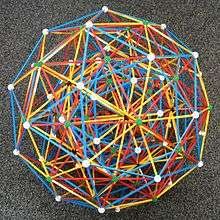Zome model of the E8 root system, projected into three-space, and represented by the vertices of the 421 polytope,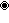A root system of rank r is a particular finite configuration of vectors, called roots, which span an r-dimensional Euclidean space and satisfy certain geometrical properties. In particular, the root system must be invariant under reflection through the hyperplane perpendicular to any root.

The E8 root system is a rank 8 root system containing 240 root vectors spanning R8. It is irreducible in the sense that it cannot be built from root systems of smaller rank. All the root vectors in E8 have the same length. It is convenient for a number of purposes to normalize them to have length √2. These 240 vectors are the vertices of a semi-regular polytope discovered by Thorold Gosset in 1900, sometimes known as the 421 polytope.

### Construction

In the so-called even coordinate system, E8 is given as the set of all vectors in R8 with length squared equal to 2 such that coordinates are either all integers or all half-integers and the sum of the coordinates is even.

Explicitly, there are 112 roots with integer entries obtained fromby taking an arbitrary combination of signs and an arbitrary permutation of coordinates, and 128 roots with half-integer entries obtained fromby taking an even number of minus signs (or, equivalently, requiring that the sum of all the eight coordinates be even). There are 240 roots in all.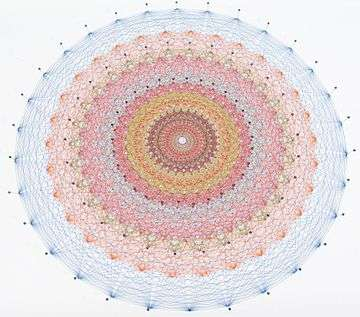The 112 roots with integer entries form a D8 root system. The E8 root system also contains a copy of A8 (which has 72 roots) as well as E6 and E7 (in fact, the latter two are usually defined as subsets of E8).

In the odd coordinate system, E8 is given by taking the roots in the even coordinate system and changing the sign of any one coordinate. The roots with integer entries are the same while those with half-integer entries have an odd number of minus signs rather than an even number.

### Dynkin diagram

The Dynkin diagram for E8 is given by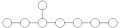.

This diagram gives a concise visual summary of the root structure. Each node of this diagram represents a simple root. A line joining two simple roots indicates that they are at an angle of 120° to each other. Two simple roots which are not joined by a line are orthogonal.

### Cartan matrix

The Cartan matrix of a rank r root system is an r × r matrix whose entries are derived from the simple roots. Specifically, the entries of the Cartan matrix are given bywhere (−,−) is the Euclidean inner product and αi are the simple roots. The entries are independent of the choice of simple roots (up to ordering).

The Cartan matrix for E8 is given byThe determinant of this matrix is equal to 1.

### Simple roots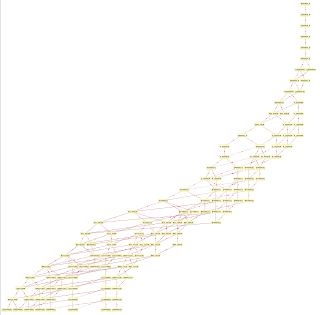Hasse diagram of E8 root poset with edge labels identifying added simple root position.

A set of simple roots for a root system Φ is a set of roots that form a basis for the Euclidean space spanned by Φ with the special property that each root has components with respect to this basis that are either all nonnegative or all nonpositive.

Given the E8 Cartan matrix (above) and a Dynkin diagram node ordering of: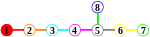One choice of simple roots is given by the rows of the following matrix:### Weyl group

The Weyl group of E8 is of order 696729600, and can be described as O+
8
(2): it is of the form 2.G.2 (that is, a stem extension by the cyclic group of order 2 of an extension of the cyclic group of order 2 by a group G) where G is the unique simple group of order 174182400 (which can be described as PSΩ8+(2)).

### E8 root lattice

Main article: E8 lattice

The integral span of the E8 root system forms a lattice in R8 naturally called the E8 root lattice. This lattice is rather remarkable in that it is the only (nontrivial) even, unimodular lattice with rank less than 16.

### Simple subalgebras of E8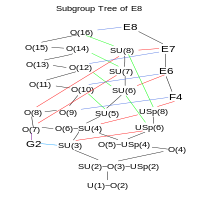An incomplete simple subgroup tree of E8

The Lie algebra E8 contains as subalgebras all the exceptional Lie algebras as well as many other important Lie algebras in mathematics and physics. The height of the Lie algebra on the diagram approximately corresponds to the rank of the algebra. A line from an algebra down to a lower algebra indicates that the lower algebra is a subalgebra of the higher algebra.

## Chevalley groups of type E8

Chevalley (1955) showed that the points of the (split) algebraic group E8 (see above) over a finite field with q elements form a finite Chevalley group, generally written E8(q), which is simple for any q, and constitutes one of the infinite families addressed by the classification of finite simple groups. Its number of elements is given by the formula (sequence A008868 in the OEIS):The first term in this sequence, the order of E8(2), namely 337804753143634806261388190614085595079991692242467651576160959909068800000 ≈ 3.38×1074, is already larger than the size of the Monster group. This group E8(2) is the last one described (but without its character table) in the ATLAS of Finite Groups.

The Schur multiplier of E8(q) is trivial, and its outer automorphism group is that of field automorphisms (i.e., cyclic of order f if q=pf where p is prime).

Lusztig (1979) described the unipotent representations of finite groups of type E8.

## Subgroups

The smaller exceptional groups E7 and E6 sit inside E8. In the compact group, both E7×SU(2)/(−1,−1) and E6×SU(3)/(Z/3Z) are maximal subgroups of E8.

The 248-dimensional adjoint representation of E8 may be considered in terms of its restricted representation to the first of these subgroups. It transforms under E7×SU(2) as a sum of tensor product representations, which may be labelled as a pair of dimensions as (3,1) + (1,133) + (2,56) (since there is a quotient in the product, these notations may strictly be taken as indicating the infinitesimal (Lie algebra) representations). Since the adjoint representation can be described by the roots together with the generators in the Cartan subalgebra, we may see that decomposition by looking at these. In this description,

• (3,1) consists of the roots (0,0,0,0,0,0,1,−1), (0,0,0,0,0,0,−1,1) and the Cartan generator corresponding to the last dimension;
• (1,133) consists of all roots with (1,1), (−1,−1), (0,0), (−½,−½) or (½,½) in the last two dimensions, together with the Cartan generators corresponding to the first seven dimensions;
• (2,56) consists of all roots with permutations of (1,0), (−1,0) or (½,−½) in the last two dimensions.

The 248-dimensional adjoint representation of E8, when similarly restricted, transforms under E6×SU(3) as: (8,1) + (1,78) + (3,27) + (3,27). We may again see the decomposition by looking at the roots together with the generators in the Cartan subalgebra. In this description,

• (8,1) consists of the roots with permutations of (1,−1,0) in the last three dimensions, together with the Cartan generator corresponding to the last two dimensions;
• (1,78) consists of all roots with (0,0,0), (−½,−½,−½) or (½,½,½) in the last three dimensions, together with the Cartan generators corresponding to the first six dimensions;
• (3,27) consists of all roots with permutations of (1,0,0), (1,1,0) or (−½,½,½) in the last three dimensions.
• (3,27) consists of all roots with permutations of (−1,0,0), (−1,−1,0) or (½,−½,−½) in the last three dimensions.

The finite quasisimple groups that can embed in (the compact form of) E8 were found by Griess & Ryba (1999).

The Dempwolff group is a subgroup of (the compact form of) E8. It is contained in the Thompson sporadic group, which acts on the underlying vector space of the Lie group E8 but does not preserve the Lie bracket. The Thompson group fixes a lattice and does preserve the Lie bracket of this lattice mod 3, giving an embedding of the Thompson group into E8(F3).

## Applications

The E8 Lie group has applications in theoretical physics and especially in string theory and supergravity. E8×E8 is the gauge group of one of the two types of heterotic string and is one of two anomaly-free gauge groups that can be coupled to the N = 1 supergravity in ten dimensions. E8 is the U-duality group of supergravity on an eight-torus (in its split form).

One way to incorporate the standard model of particle physics into heterotic string theory is the symmetry breaking of E8 to its maximal subalgebra SU(3)×E6.

In 1982, Michael Freedman used the E8 lattice to construct an example of a topological 4-manifold, the E8 manifold, which has no smooth structure.

Antony Garrett Lisi's incomplete "An Exceptionally Simple Theory of Everything" attempts to describe all known fundamental interactions in physics as part of the E8 Lie algebra.

R. Coldea, D. A. Tennant, and E. M. Wheeler et al. (2010) reported an experiment where the electron spins of a cobalt-niobium crystal exhibited, under certain conditions, two of the eight peaks related to E8 that were predicted by Zamolodchikov (1989).

## Notes

1. Платонов, Владимир П.; Рапинчук, Андрей С. (1991), Алгебраические группы и теория чисел, Наука, ISBN 5-02-014191-7 (English translation: Platonov, Vladimir P.; Rapinchuk, Andrei S. (1994), Algebraic groups and number theory, Academic Press, ISBN 0-12-558180-7), §2.2.4
2. Conway, John Horton; Curtis, Robert Turner; Norton, Simon Phillips; Parker, Richard A; Wilson, Robert Arnott (1985), Atlas of Finite Groups: Maximal Subgroups and Ordinary Characters for Simple Groups, Oxford University Press, p. 85, ISBN 0-19-853199-0
3. Carter, Roger W. (1989), Simple Groups of Lie Type, Wiley Classics Library, John Wiley & Sons, ISBN 0-471-50683-4
4. Wilson, Robert A. (2009), The Finite Simple Groups, Graduate Texts in Mathematics, 251, Springer-Verlag, ISBN 1-84800-987-9
5. Conway &al, op. cit., p. 235.
6. A. G. Lisi; J. O. Weatherall (2010). "A Geometric Theory of Everything". Scientific American. 303 (6): 54–61. doi:10.1038/scientificamerican1210-54. PMID 21141358.
7. Greg Boustead (2008-11-17). "Garrett Lisi's Exceptional Approach to Everything". SEED Magazine.
8. Most beautiful math structure appears in lab for first time, New Scientist, January 2010 (retrieved January 8, 2010).